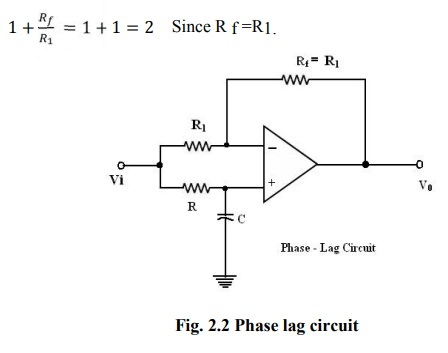Home | | Linear Integrated Circuits | Phase Shift Circuits

# Phase Shift Circuits

The phase shift circuits produce phase shifts that depend on the frequency and maintain a constant gain.

Phase Shift Circuits

The phase shift circuits produce phase shifts that depend on the frequency and maintain a constant gain. These circuits are also called constant-delay filters or all-pass filters. That constant delay refers to the fact the time difference between input and output remains constant when frequency is changed over a range of operating frequencies.

This is called all-pass because normally a constant gain is maintained for all the frequencies within the operating range. The two types of circuits, for lagging phase angles and leading phase angles.

## Phase-lag circuit:

Phase log circuit is constructed using an op-amp, connected in both inverting and non inverting modes. To analyze the circuit operation, it is assumed that the input voltage v1 drives a simple inverting amplifier with inverting input applied at(-)terminal of op-amp and a non inverting amplifier with a low-pass filter.

It is also assumed that inverting gain is -1 and non-inverting gain after the low-pass circuit  isThe relationship is complex as defined above equation and it shows that it has both magnitude and phase. Since the numerator and denominator are complex conjugates, their magnitudes are identical and the overall phase angle equals the angle of numerator less the angle of the denominator.